SSC CGL Previous Year Questions: Simple & Compound Interest - 2

# SSC CGL Previous Year Questions: Simple & Compound Interest - 2 - SSC CGL

Test Description

## 41 Questions MCQ Test SSC CGL (Tier - 1) - Previous Year Papers (Topic Wise) - SSC CGL Previous Year Questions: Simple & Compound Interest - 2

SSC CGL Previous Year Questions: Simple & Compound Interest - 2 for SSC CGL 2023 is part of SSC CGL (Tier - 1) - Previous Year Papers (Topic Wise) preparation. The SSC CGL Previous Year Questions: Simple & Compound Interest - 2 questions and answers have been prepared according to the SSC CGL exam syllabus.The SSC CGL Previous Year Questions: Simple & Compound Interest - 2 MCQs are made for SSC CGL 2023 Exam. Find important definitions, questions, notes, meanings, examples, exercises, MCQs and online tests for SSC CGL Previous Year Questions: Simple & Compound Interest - 2 below.
Solutions of SSC CGL Previous Year Questions: Simple & Compound Interest - 2 questions in English are available as part of our SSC CGL (Tier - 1) - Previous Year Papers (Topic Wise) for SSC CGL & SSC CGL Previous Year Questions: Simple & Compound Interest - 2 solutions in Hindi for SSC CGL (Tier - 1) - Previous Year Papers (Topic Wise) course. Download more important topics, notes, lectures and mock test series for SSC CGL Exam by signing up for free. Attempt SSC CGL Previous Year Questions: Simple & Compound Interest - 2 | 41 questions in 41 minutes | Mock test for SSC CGL preparation | Free important questions MCQ to study SSC CGL (Tier - 1) - Previous Year Papers (Topic Wise) for SSC CGL Exam | Download free PDF with solutions
 1 Crore+ students have signed up on EduRev. Have you?
SSC CGL Previous Year Questions: Simple & Compound Interest - 2 - Question 1

### In certain years a sum of money is doubled itself at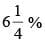simple interest per annum, then the required time will be  (SSC CGL 1st Sit. 2015)

Detailed Solution for SSC CGL Previous Year Questions: Simple & Compound Interest - 2 - Question 1

Let x be the principal amount ‘y’ be the time to double the money.
Then interest will also be ‘x’.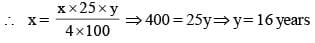SSC CGL Previous Year Questions: Simple & Compound Interest - 2 - Question 2

### The population of a town increases by 5% every year. If the present population is 9261, the population 3 years ago was    (SSC CGL 1st Sit. 2015)

Detailed Solution for SSC CGL Previous Year Questions: Simple & Compound Interest - 2 - Question 2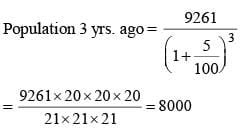SSC CGL Previous Year Questions: Simple & Compound Interest - 2 - Question 3

### The income of a company increases 20% per annum. If its income is ₹ 26,64,000 in the year 2012, then its income in the year 2010 was:    (SSC CHSL 2015)

Detailed Solution for SSC CGL Previous Year Questions: Simple & Compound Interest - 2 - Question 3

Income in 2012 = ₹ 26, 64,000
Every year % of increase in income = 20%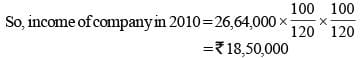SSC CGL Previous Year Questions: Simple & Compound Interest - 2 - Question 4

The compound interest on ₹ 12000 for 9 months at 20% per annum, interest being compounded quarterly is:    (SSC CHSL 2015)

Detailed Solution for SSC CGL Previous Year Questions: Simple & Compound Interest - 2 - Question 4

P = ₹ 12000; R = 20% per annum  = 5% per quarter T = 9 months  = 3 quarters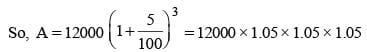= ₹ 13891.1
So, CI = 13891.10  – 12000  = ₹ 1891.10

SSC CGL Previous Year Questions: Simple & Compound Interest - 2 - Question 5

A certain amount of money earns ₹ 540 as Simple Interest in 3 years. If it earns a Compound Interest of  ₹ 376.20 at the same rate of interest in 2 years, find the amount. (in rupees)  (SSC Sub. Ins. 2015)

Detailed Solution for SSC CGL Previous Year Questions: Simple & Compound Interest - 2 - Question 5

Let amount is P, rate is r and time is t.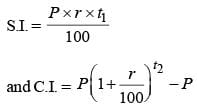from question, we have
S.I. = ₹ 540, C.I. = ₹ 376.20
t1 = 3 years, t2 = 2 years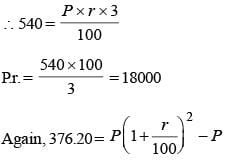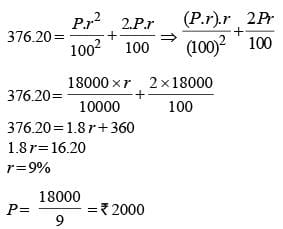SSC CGL Previous Year Questions: Simple & Compound Interest - 2 - Question 6

A sum of ₹ 210 was taken as a loan. This is to be paid back in two equal instalments. If the rate of interest be 10% compounded annually, then the value of each instalment is    (SSC CHSL 2014)

Detailed Solution for SSC CGL Previous Year Questions: Simple & Compound Interest - 2 - Question 6

Let each installment be x.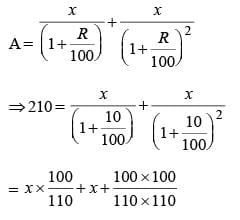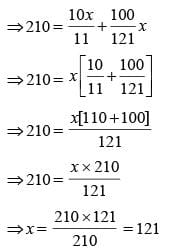SSC CGL Previous Year Questions: Simple & Compound Interest - 2 - Question 7

The population of a village increases by 5% annually. If its present population is 4410, then its population 2 years ago was    (SSC CHSL 2014)

Detailed Solution for SSC CGL Previous Year Questions: Simple & Compound Interest - 2 - Question 7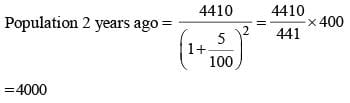SSC CGL Previous Year Questions: Simple & Compound Interest - 2 - Question 8

Rekha invested a sum of ₹ 12000 at 5% per annum compound interest. She received an amount of ₹ 13230 after n years.
Find n.    (SSC Sub. Ins. 2014)

Detailed Solution for SSC CGL Previous Year Questions: Simple & Compound Interest - 2 - Question 8

P = ₹ 12000, Rate = 5 %, Time (n) = ?,  Amount = 13230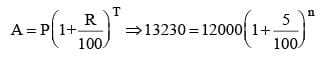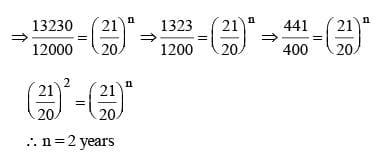SSC CGL Previous Year Questions: Simple & Compound Interest - 2 - Question 9

A sum amounts double in 8 years by simple interest. Then the rate of simple interest p.a. is    (SSC Sub. Ins. 2014)

Detailed Solution for SSC CGL Previous Year Questions: Simple & Compound Interest - 2 - Question 9

Let P be the principle amount and R be rate of interest.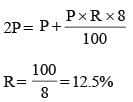SSC CGL Previous Year Questions: Simple & Compound Interest - 2 - Question 10

Ram borrows a certain sum of money at 8% per annum simple interest and Rahim borrows ₹ 2,000 at 5% per annum simple interest. If the interest at the end of 3 years is equal, then the amount borrowed by Ram is    (SSC Multitasking 2014)

Detailed Solution for SSC CGL Previous Year Questions: Simple & Compound Interest - 2 - Question 10

Let Ram borrowed  ₹ P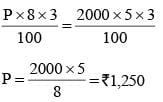SSC CGL Previous Year Questions: Simple & Compound Interest - 2 - Question 11

A man borrowed some money from a private organisation at 5% simple interest per annum. He lended 50% of this money to another person at 10% compound interest per annum and thereby the man made a profit of ₹ 3205 in 4 years. The man borrowed    (SSC CGL 2nd Sit. 2014)

Detailed Solution for SSC CGL Previous Year Questions: Simple & Compound Interest - 2 - Question 11

Let the required amount = P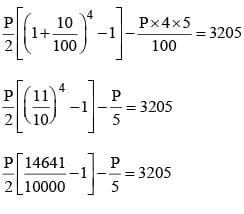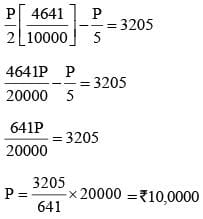SSC CGL Previous Year Questions: Simple & Compound Interest - 2 - Question 12

X borrowed some money from a source at 8% simple interest and lent it to Y at 12% simple interest on the same day and gained ₹ 4,800 after 3 years. The amount X borrowed, in ₹, is    (SSC Multitasking 2014)

Detailed Solution for SSC CGL Previous Year Questions: Simple & Compound Interest - 2 - Question 12

Let X borrow  ₹ P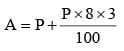Total amount x get from y, A' =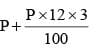According to question
A' – A = 4800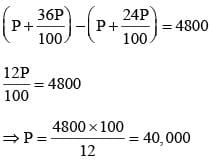SSC CGL Previous Year Questions: Simple & Compound Interest - 2 - Question 13

If the compound interest on a certain sum for two years at 12% per annum is ₹ 2,544, the simple interest on it at the same rate for 2 years will be    (SSC CGL 2nd Sit. 2013)

Detailed Solution for SSC CGL Previous Year Questions: Simple & Compound Interest - 2 - Question 13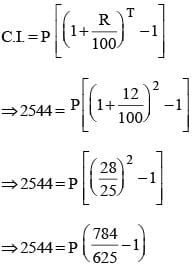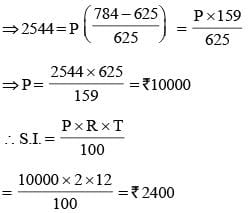SSC CGL Previous Year Questions: Simple & Compound Interest - 2 - Question 14

Two equal sums were borrowed at 8% simple interest per annum for 2 years and 3 years respectively. The difference in the interest was ₹ 56. The sums borrowed were    (SSC CGL 2nd Sit. 2013)

Detailed Solution for SSC CGL Previous Year Questions: Simple & Compound Interest - 2 - Question 14

Let principal be represented by P.
Ist Case: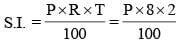IInd Case :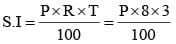According to question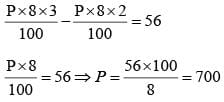SSC CGL Previous Year Questions: Simple & Compound Interest - 2 - Question 15

The simple interest an ₹ 4,000 in 3 years at the rate of x% per annum equals the simple interest on ₹ 5,000 at the rate of 12% per annum in 2 years. The value of x is    (SSC CGL 2nd Sit. 2013)

Detailed Solution for SSC CGL Previous Year Questions: Simple & Compound Interest - 2 - Question 15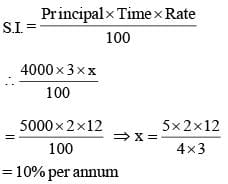SSC CGL Previous Year Questions: Simple & Compound Interest - 2 - Question 16

There is 100% increase to an amount in 8 years, at simple interest. Find the compound interest of ₹ 8000 after 2 years at the same rate of interest.    (SSC CGL 1st Sit. 2013)

Detailed Solution for SSC CGL Previous Year Questions: Simple & Compound Interest - 2 - Question 16

Principal = ₹ 8000
SI = 8000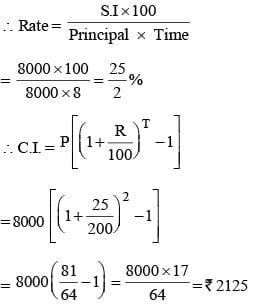SSC CGL Previous Year Questions: Simple & Compound Interest - 2 - Question 17

The interest on a certain sum of money is ₹ 22 and the true discount on the same sum for the same time and at the same rate is ₹ 20, find the sum.   (SSC CGL 1st Sit. 2013)

Detailed Solution for SSC CGL Previous Year Questions: Simple & Compound Interest - 2 - Question 17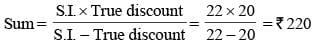SSC CGL Previous Year Questions: Simple & Compound Interest - 2 - Question 18

The time in which ₹ 80,000 amounts to ₹ 92,610 at 10% p.a. at compound interest, interest being compounded semi annually is:    (SSC CGL 1st Sit. 2013)

Detailed Solution for SSC CGL Previous Year Questions: Simple & Compound Interest - 2 - Question 18

Time = T half year = R = 5% per half year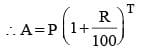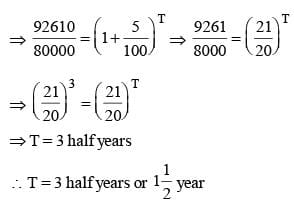SSC CGL Previous Year Questions: Simple & Compound Interest - 2 - Question 19

The value of a machine depreciates every year by 10%. if its present value is ₹ 50,000 then the value of the machine after 2 years is __________.    (SSC CGL 1st Sit. 2013)

Detailed Solution for SSC CGL Previous Year Questions: Simple & Compound Interest - 2 - Question 19

Depreciated value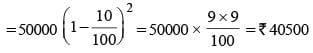SSC CGL Previous Year Questions: Simple & Compound Interest - 2 - Question 20

A sum of money placed at compound interest doubles itself in 5 years. It will amount to eight times of itself in:    (SSC Sub. Ins. 2013)

Detailed Solution for SSC CGL Previous Year Questions: Simple & Compound Interest - 2 - Question 20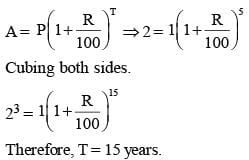SSC CGL Previous Year Questions: Simple & Compound Interest - 2 - Question 21

A person deposited ₹ 500 for 4 years and ₹ 600 for 3 years at the same rate of simple interest in a bank. Altogether he received ₹ 190 as interest. The rate of simple interest per annum was    (SSC Multitasking 2013)

Detailed Solution for SSC CGL Previous Year Questions: Simple & Compound Interest - 2 - Question 21

S.I (Simple Interest)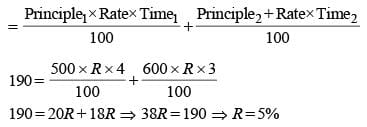SSC CGL Previous Year Questions: Simple & Compound Interest - 2 - Question 22

A sum of money becomes 1.331 times in 3 years as compound interest. The rate of interest is    (SSC Multitasking 2013)

Detailed Solution for SSC CGL Previous Year Questions: Simple & Compound Interest - 2 - Question 22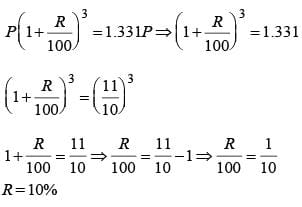SSC CGL Previous Year Questions: Simple & Compound Interest - 2 - Question 23

A sum becomes ₹ 2,916 in 2 years at 8% per annum compound interest. The simple interest at 9% per annum for 3 years on the same amount will be   (SSC CHSL 2013)

Detailed Solution for SSC CGL Previous Year Questions: Simple & Compound Interest - 2 - Question 23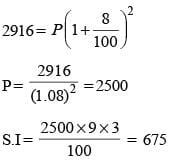SSC CGL Previous Year Questions: Simple & Compound Interest - 2 - Question 24

The difference between the interests received from two different banks on ₹ 500 for 2 years is ₹ 2.50. The difference between their rates is:    (SSC CHSL 2012)

Detailed Solution for SSC CGL Previous Year Questions: Simple & Compound Interest - 2 - Question 24

Let r1 and r2 are the rates of interests.
So, the difference in S.I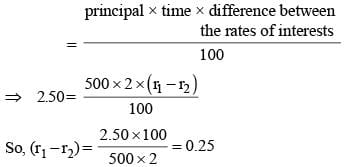SSC CGL Previous Year Questions: Simple & Compound Interest - 2 - Question 25

A principal of ₹ 10,000, after 2 years compounded annually, the rate of interest being 10% per annum during the first year and 12% per annum during the second year (in rupees) will amount to:    (SSC CHSL 2012)

Detailed Solution for SSC CGL Previous Year Questions: Simple & Compound Interest - 2 - Question 25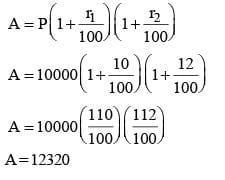SSC CGL Previous Year Questions: Simple & Compound Interest - 2 - Question 26

The difference between C. I. (Compound Interest) and S.I. (Simple Interest) on a sum of ₹ 4,000 for 2 years at 5% p.a. payable yearly is (SSC CGL 2nd Sit. 2012)

Detailed Solution for SSC CGL Previous Year Questions: Simple & Compound Interest - 2 - Question 26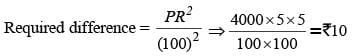SSC CGL Previous Year Questions: Simple & Compound Interest - 2 - Question 27

A person takes a loan of ₹10,000 partly from a bank at 8% p.a. and remaining from another bank at 10% p.a. He pays a total interest of ₹ 950 per annum. Amount of loan taken from the first bank (in ₹) is (SSC CGL 1st Sit. 2012)

Detailed Solution for SSC CGL Previous Year Questions: Simple & Compound Interest - 2 - Question 27

If the amount of loan taken from the first bank be ₹ x, then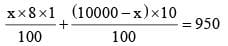⇒ 8x + 100000 – 10x = 95000
⇒ 2x = 100000 – 95000 = 5000
⇒ x = ₹ 2500
Alternate Method: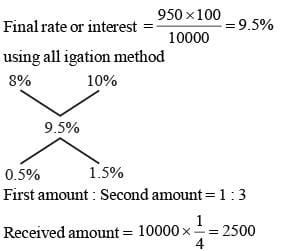SSC CGL Previous Year Questions: Simple & Compound Interest - 2 - Question 28

Prabhat look a certain amount as a loan from a bank at the rate of 8% p.a. simple interest and gave the same amount to Ashish as a loan at the rate of 12% p.a. If at the end of 12 years, he made a profit of ₹ 960 in the deal, then the original amount was:    (SSC CGL 1st Sit. 2012)

Detailed Solution for SSC CGL Previous Year Questions: Simple & Compound Interest - 2 - Question 28

Difference of rates = 4%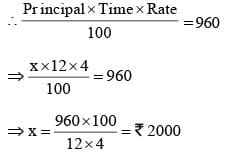SSC CGL Previous Year Questions: Simple & Compound Interest - 2 - Question 29

If  ₹5,000 becomes ₹5,700 in a year's time, what will ₹7,000 become at the end of 5 years at the same rate of simple interest?    (SSC CGL 1st Sit. 2012)

Detailed Solution for SSC CGL Previous Year Questions: Simple & Compound Interest - 2 - Question 29

Interest = 5700 – 5000 = ₹ 700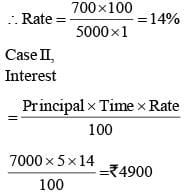Amount = 7000 + 4900 = ₹ 11900

SSC CGL Previous Year Questions: Simple & Compound Interest - 2 - Question 30

The difference between the compound interest and simple interest for the amount ₹ 5,000 in 2 years is ₹ 32. The rate of interest is    (SSC CGL 2nd Sit. 2011)

Detailed Solution for SSC CGL Previous Year Questions: Simple & Compound Interest - 2 - Question 30

Difference of 2 years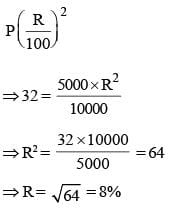SSC CGL Previous Year Questions: Simple & Compound Interest - 2 - Question 31

Simple interest on a certain sum for 6 years is 9/25 of the sum. The rate of interest is    (SSC CGL 2nd Sit. 2011)

Detailed Solution for SSC CGL Previous Year Questions: Simple & Compound Interest - 2 - Question 31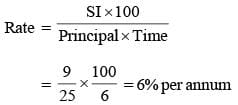SSC CGL Previous Year Questions: Simple & Compound Interest - 2 - Question 32

A sum of ₹ 12,000 deposited at compound interest becomes double after 5 years. After 20 years, it will become   (SSC CGL 2nd Sit. 2011)

Detailed Solution for SSC CGL Previous Year Questions: Simple & Compound Interest - 2 - Question 32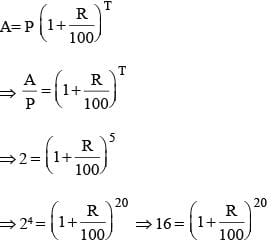Hence, the principal will become 16 times i.e. ₹ (16 × 12000)
= ₹192000

SSC CGL Previous Year Questions: Simple & Compound Interest - 2 - Question 33

The difference between the compound interest and simple interest on ₹ 10,000 for 2 years is ₹ 25. The rate of interest per annum is    (SSC CGL 2nd Sit. 2011)

Detailed Solution for SSC CGL Previous Year Questions: Simple & Compound Interest - 2 - Question 33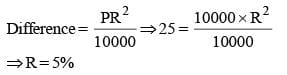SSC CGL Previous Year Questions: Simple & Compound Interest - 2 - Question 34

The simple interest on a sum for 5 years is one fourth of the sum. The rate of interest per annum is    (SSC CGL 1st Sit. 2011)

Detailed Solution for SSC CGL Previous Year Questions: Simple & Compound Interest - 2 - Question 34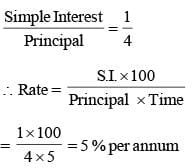SSC CGL Previous Year Questions: Simple & Compound Interest - 2 - Question 35

A sum of money placed at compound interest doubles itself in 4 years. In how many years will it amount to four times itself?    (SSC CGL 1st Sit. 2011)

Detailed Solution for SSC CGL Previous Year Questions: Simple & Compound Interest - 2 - Question 35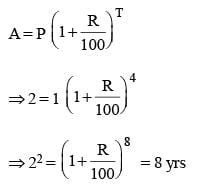SSC CGL Previous Year Questions: Simple & Compound Interest - 2 - Question 36

A sum of ₹ 12,000, deposited at compound interest becomes double after 5 years. How much will it be after 20 years?    (SSC CGL 2nd Sit. 2010)

Detailed Solution for SSC CGL Previous Year Questions: Simple & Compound Interest - 2 - Question 36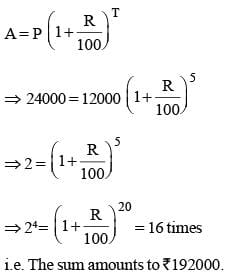SSC CGL Previous Year Questions: Simple & Compound Interest - 2 - Question 37

In how many years will a sum of money double itself at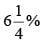simple interest per annum?   (SSC CGL 2nd Sit. 2010)

Detailed Solution for SSC CGL Previous Year Questions: Simple & Compound Interest - 2 - Question 37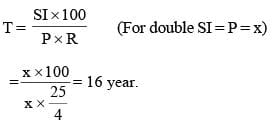SSC CGL Previous Year Questions: Simple & Compound Interest - 2 - Question 38

In how many years will a sum of ₹ 800 at 10% per annum compound interest, compounded semi-annually becomes ₹ 926.10?    (SSC CGL 2nd Sit. 2010)

Detailed Solution for SSC CGL Previous Year Questions: Simple & Compound Interest - 2 - Question 38

Rate = 10% per annum = 5% half yearly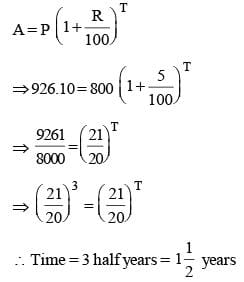SSC CGL Previous Year Questions: Simple & Compound Interest - 2 - Question 39

What annual payment will discharge a debt of ₹6,450 due in 4 years at 5% per annum simple interest?    (SSC CGL 1st Sit. 2010)

Detailed Solution for SSC CGL Previous Year Questions: Simple & Compound Interest - 2 - Question 39

Let the annual instalment be ₹ x.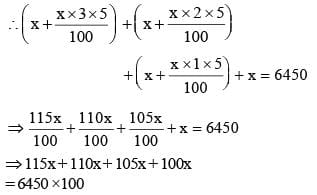SSC CGL Previous Year Questions: Simple & Compound Interest - 2 - Question 40

At what rate percent per annum will a sum of ₹ 1,000 amount to ₹ 1,102.50 in 2 years at compound interest?   (SSC CGL 1st Sit. 2010)

Detailed Solution for SSC CGL Previous Year Questions: Simple & Compound Interest - 2 - Question 40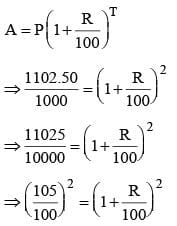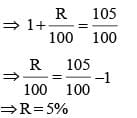SSC CGL Previous Year Questions: Simple & Compound Interest - 2 - Question 41

A sum of money at compound interest doubles itself in 15 years. It will become eight times of itself in    (SSC CGL 1st Sit. 2010)

Detailed Solution for SSC CGL Previous Year Questions: Simple & Compound Interest - 2 - Question 41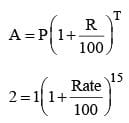Cubing on both sides, we have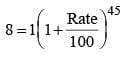Required time = 45 years

## SSC CGL (Tier - 1) - Previous Year Papers (Topic Wise)

250 tests
Information about SSC CGL Previous Year Questions: Simple & Compound Interest - 2 Page
In this test you can find the Exam questions for SSC CGL Previous Year Questions: Simple & Compound Interest - 2 solved & explained in the simplest way possible. Besides giving Questions and answers for SSC CGL Previous Year Questions: Simple & Compound Interest - 2, EduRev gives you an ample number of Online tests for practice

250 tests